# Respiratory and Motion Artefacts Removal from ICG Signal Using Denoising Techniques for Hemodynamic Parameters Monitoring

Respiratory and Motion Artefacts Removal from ICG Signal Using Denoising Techniques for Hemodynamic Parameters Monitoring
Biomedical Engineering Laboratory, Faculty of Technology, Tlemcen University, Tlemcen 13000, Algeria

Corresponding Author Email:
Page:
919-928
|
DOI:
https://doi.org/10.18280/ts.380401
11 July 2021
|
Accepted:
18 August 2021
|
Published:
31 August 2021
| Citation

OPEN ACCESS

Abstract:

The impedance cardiography (ICG) is a reliable, non-invasive method widely used in clinical practice for the measurement of a multitude of hemodynamic parameters for the diagnosis of cardiovascular disease and continuous monitoring. Signal processing field is necessary to eliminate noises as an artefact of respiration and movement, to extract features characteristics from ICG signals. This paper discusses the concept of wavelet denoising based on scale-dependent thresholding, which is used in two types of the orthogonal wavelet family: Daubechies wavelets (db) and Symlet (sym) applied to the ICG. The study is based on wavelet coefficients that are thresholded using Sureshrink, NeighBlock, and classical thresholds such as Rigrsure and Sqtwolog; they are all compared with linear filters as well as with the LMS-based adaptive filtering algorithm already implemented in biosignal denoising. The results of the evaluation of the performance parameters show that the best denoising technique that gives good results in noise reduction is that of sym8 wavelets at level 5, and the most optimal thresholding technique is the Rigrsure technique with a mean error rate (MER) equal to 0.0001%. The proposed method has shown the reliability of results that can help us later to extract precisely significant information to diagnose earlier and monitor cardiovascular disorders.

Keywords:

impedance cardiography, orthogonal wavelet, thresholding technique, linear filter, adaptive filter, biosignal denoising

1. Introduction

ICG is widely used in clinical applications, such as hypertension, surgery, cardiovascular disease and pregnancy . It emerges in 1940, it is simple, safe, easy-to-apply, cost-effective, non-invasive method of diagnosis and medical monitoring that measures the change in blood volume due to impedance changes using a system of electrodes placed on the patient's skin. The detected impedance waveform called ICG is vulnerable to noise such as respiratory and motion artifacts due to patient movement during acquisition, poor electrode placement and electrode material. The essential step in deriving several significant parameters is the analysis of this type of waveform using the concept of noise cancellation. Some researchers have applied algorithms such as the LMS-based adaptive filter [2, 3] as well as linear filters such as Elliptic and Butterworth  to remove noise and artifacts from the ICG waveform.

However, wavelet analysis is an advanced signal processing tool, although its mathematical foundations date back to Joseph Fourier in the 19th century, when the researcher established the foundations for the Theory of Frequency Analysis . It is a method that measures average fluctuations at different scales that have shown a significant reduction in noise and preserves the characteristics of the signal. In 1909 the wavelet was discussed in Alfred Haar's thesis. Afterwards, these methods of analysis were developed by Meyer and other researchers . In signal analysis, the wavelet transforms the signal to extract the relevant information after noise suppression . One of the discrete wavelets is the sym26 which is used for noise suppression of the ICG signal .

Five methods of denoising are compared using different filters: the Savitzky-Golay filter compared to the median filter, the bandpass filter, the wavelet (db8) and the moving average filter. The results show that the first filter is the best . A comparison between the Empirical Ensemble Empirical Mode Decomposition (EEMD), the optimal FIR filter, and the Symlet (sym8) wavelet family for ICG denoising where the latter is better . Choudhari's study has shown that db4 is the most efficient for denoising . Chabchoub argued that the best level of wavelet decay is the one that gives the greatest softness separation between signal and noise; he found that the db8 wavelet family is better than the other wavelet families . According to Chabchoub , and other papers in the scientific literature [8-10, 12], the best denoising method has the highest signal-to-noise ratio, the lowest root mean square error, the smallest percentage difference, and the smallest reconstructed error. The ICG denoising process is therefore necessary to pass through the multi-scale decomposition, then the threshold coefficients, after which the signal will be reconstructed, using the inverse of the Discrete Wave Transformation (DWT).

There is no better universal standard threshold applied for the determination technique, which is why there are multiple threshold techniques such as classical thresholding, SureShrink and Neigh-Block. These techniques have provided reliable results for denoising the ECG signal and have never been used for denoising the ICG signal . The advantage of wavelet denoising is that it preserves the signal characteristics and eliminates noise at all frequencies. However, it is different from the smoothing used to suppress high frequencies and maintain low frequencies .

This study aims to study the denoising of the ICG signal using linear filters. In addition, the LMS adaptive filter tested, as well as different wavelet thresholding techniques such as SureShrink (proposed by Donoho and Johnstone , NeighBlock (local thresholding), Rigrsure and Sqtwolog (universal thresholding) are compared.

2. Material and Methods

2.1 The impedance cardiography (ICG) method

Research on the impedance technique of cardiography is devoted to the evaluation of the structural configurations and physiological activities of biological tissues; this technique measures the variations in Z impedance in the thorax during the cardiac cycle. Studies in this field are applied to the diagnosis and monitoring of the patient's pathological state and to the detection of cardiovascular disorders through the calculation of hemodynamic parameters such as systolic volume and cardiac output for the elderly , by applying a weak electric field longitudinally from 0.2 mA to 5 mA, and of low frequency from 50 kHz to 100 kHz across a segment of the thorax with the Tetrapolar technique . Figure 1a shows the electrode configuration of the ICG technique with the outer electrode for current injection and the inner electrode for impedance measurement values during diastole.

Figure 1b shows the typical ICG signal and the ECG signal tracing.

## 1.png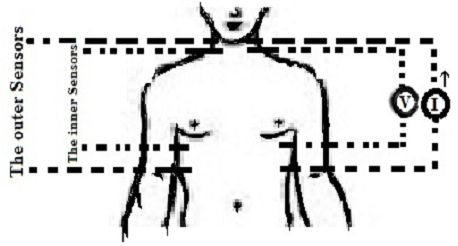(a) Electrode configuration of the ICG technique

## 2.png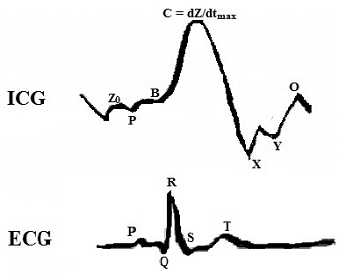(b) Typical ICG signal and ECG signal tracing

Figure 1. The ICG signal measurement

The ICG signal is used to calculate cardiac function parameters  such as stroke volume (SV) Eq. (1), New Bern Stein [18, 19], Cardiac output (CO) Eq. (2) is an effective parameter used to evaluate the mechanical activities of the heart and cardiovascular disorders.

$S V=V_{c} \sqrt{L V E T *\left(\frac{1}{Z_{0}}\right)\left(\frac{d Z}{d t}\right) \max }$     (1)

$C O=H R * S V$      (2)

where, $V_{c}$ is the intrathoracic blood volume expressed in mL, and LVET is the left ventricle ejection time, Z0: basic thoracic impedance, (dZ/ dt)max is maximum aortic flow rate and presents the maximum on the ICG signal curve, HR is the heart rate.

2.1.1 ICG signal

The ICG waveform range is 0.8 to 20 Hz. This is the first derivative of Z (dZ/dt) shown in the Figure 1b. It is characterised by points, where, Z0: basic thoracic impedance varies from 20 to 33Ω and 27 to 48 Ω for men and women respectively ; P or A: atrial wave; B: aortic valve opening; C: maximum aortic flow rate (dZ/ dt)max; X: aortic valve closure; Y: the pulmonary valve closing; O: mitral valve opening. These points are detected to extract indices such as SV and CO, as well as characteristic time intervals such as left ventricular ejection time (LVET) to diagnose cardiovascular disease . The advantage of this technique is that it is simple to perform, quickly to respond to rhythm, less expensive, non-invasive and safe, and allows continuous real-time monitoring .

Many researchers have devoted their studies to the algorithms for detecting characteristic points. While the problem with acquired ICG signals is that of respiratory and motion artefacts, which have very low frequency ranges of 0.04 Hz to 2 Hz and 0.1 Hz to 10 Hz, respectively, it has nevertheless made its analysis somewhat challenging. To this end, this work aims to address this problem.

2.2 Transform wavelet

A wavelet has a short-term waveform; orthogonal wavelets better characterise the local properties of signals than Fourier bases. Its analysis is used to decompose the signal into shifted and scaled versions of the mother wavelet, whose matrix is defined as follows :

$\Psi(x)=\left\{\begin{array}{cc}1 \text { for } & 0<x<\frac{1}{2} \\ -1 \text { for } & \frac{1}{2}<x<1 \\ 0 & \text { otherwise. }\end{array}\right.$     (3)

Transformation wavelets used in a multitude of applications, mainly for feature extraction, classification and noise elimination , have simplified signal analysis. In several fields, it has become well known over the last 15 years, such as industry, medicine, where it is used for noise removal from physiological signals  such as ECG, EEG, and others.

There are two types of wavelet transformation: continuous wavelet transformation (CWT) and discrete wavelet transformation (DWT). The latter, which is concerned with statistical parameters, is precisely discretely sampled functions, it describes the frequency content of the signal at given moments, thanks to filter banks that decompose the signal into coefficients with Details, and Approximation , it is used to select scales and dynamic positions to obtain more accuracy, the DWT Eq. (4) is as follows:

$X[a, \mathrm{~B}]=\sum_{n=-\infty}^{+\infty} x[n] \Psi_{a, B}[n]$     (4)

$\psi_{a, B}[n]=\frac{1}{\sqrt{a}}\left[\psi_{(\mathrm{n}-\mathrm{B}) / \alpha}\quad\right]$     (5)

where, ɑ and ʙ are the wavelet location parameters, x[n] is the signal, n is the samples number, and ѱ(.) is the mother wavelet .

2.2.1 Wavelet thresholding

The wavelet thresholding method is used to threshold the wavelet coefficients by eliminating their noisy part . Noise-free wavelet coefficients are indeed rare, and those of low amplitude are set to zero. The name wavelet thresholding is derived from the comparison of a coefficient with a threshold to determine whether or not it is a desirable constituent part of the original signal. The wavelet decomposes the signal into approximations cᵢ, which represent the low frequencies, where most of the information in the signal resides, and details dᵢ, which represent the high frequencies.

The thresholding derives the significant coefficients of cᵢ; if they are less than a threshold level λ, they will be equal to zero. This threshold depends on the level of decomposition, which is called Sureshrink [15, 27], and an inverse discrete wavelet transformation using IDWT which leads to a less noisy reconstruction of the signal . Among these threshold methods are classical thresholding such as Rigrsure which used the Stein's unbiased risk principle (SURE), and Sqtwolog which used for the universal threshold. The methods are respectively defined as follows:

$\mathrm{th}_{\mathrm{i}}=\theta_{\mathrm{i}} \sqrt{2 \log \left(N_{\mathrm{i}}\right)}$     (6)

$\theta_{\mathrm{i}}=\frac{\operatorname{median}|\omega|}{0.6745}$     (7)

where, $\theta_{\mathrm{i}}$ is the mean absolute deviation and $\mathrm{N}_{\mathrm{i}}$ is the length of the noisy signal, and $\omega$ is the wavelet coefficient to scale $\mathrm{j}$.

$\mathrm{th}_{\mathrm{i}}=\theta_{\mathrm{i}} \sqrt{\omega_{\mathrm{a}}}$      (8)

where, $\theta$ is the standard deviation of noisy signal, and $\omega_{\mathrm{a}}$ is the coefficient wavelet square.

The Sureshrink method, proposed by Donoho and Johnstone , is based on Steins' unbiased risk estimate (SURE). Its principle is to set the coefficients to zero below a certain threshold. For each sub-band, the calculation of the threshold is crucial and is determined by the level of decomposition .

The neighbourhood block method, proposed by Cai and Silverman , is based on the calculation of the shrinkage factor within blocks of successive coefficients. It is applied to the group of adjacent coefficients, and is not applied for each coefficient and level. The use of multiple thresholds for all coefficients improves noise reduction performance . The choice of the threshold can be chosen according to the local noise levels, this technique calculates a threshold value with the neighbourhood [28, 29], and is based on the following steps :

• Step 1: Decomposition of the signal in coefficient with the DWT;
• Step 2: Carry out the coefficients in disjoint block bi,j for each level;
• Step 3: The shrinkage factor rule is chosen according to the local properties of the coefficient. It is defined in Eq. (6) as follows:

$\beta_{\mathrm{i}, \mathrm{j}}=\left(\max \left(0,1-\frac{\lambda L \theta^{2}}{S^{2}}\right)\right)$      (9)

with,

$L=L_{0}+2 L_{1}$

$L_{0}=\frac{\log (n)}{2}$

$L_{1}=\max \left(1, \frac{L_{0}}{2}\right)$

$\mathrm{S}^{2}=\sum_{j, k \in \beta i, j} \theta_{\mathrm{i}, \mathrm{j}}$

$K=1, \ldots L_{1}$

where, i is the block, j is the level, λ=4.5053, and $\theta^{2}$ is the variance of the extended block.

There are two thresholding approaches which are defined as follows :

Hard: $\mathrm{d}_{\mathrm{i}}=\left\{\begin{array}{c}d_{\mathrm{i}} \text { if }\left|d_{\mathrm{i}}\right|>\lambda \\ 0 \text { else }\end{array}\right\}$      (10)

Soft: $\mathrm{d}_{\mathrm{i}}=\left\{\begin{array}{c}d_{\mathrm{i}}-\lambda \text { for } d_{\mathrm{i}}>\lambda \\ d_{\mathrm{i}}+\lambda \text { for } d_{\mathrm{i}}<-\lambda \\ 0 \text { else }\end{array}\right\}$       (11)

Simple wavelet thresholding is the hard thresholding but soft thresholding is more efficient, considered as the wavelet denoising (shrinkage) method, i.e., a non-linear process integrated in a linear denoising technique. According to Donoho, the calculation of λ is based on Stein's unbiased risk principle (SURE) [15, 28] as follows:

$\lambda=\sqrt{2 \log M}$      (12)

where, M coefficients numbers.

2.3 Proposed methodology

2.3.1 ICG signal recordings

ICG signal samples from 10 healthy subjects were recorded with BioLab v.3.0.13 software at a sampling rate of 1000 Hz. The ICG device was implemented in tree sections comprising the Howland current injection stage, the latching amplifier stage for impedance detection and the ICG evaluation interface. ICG measurements in the tetrapolar configuration are based on four electrodes. The proposed methodology is mainly based on the denoising process, with some filtering techniques: linear filters, LMS adaptive filters and wavelets. These techniques were tested to eliminate noise and have a better visibility of the ICG waveform.

2.3.2 Linear filters method

The linear filters used to denoise the ICG signal of 10 participants are:

• Butterworth
•  Elliptical
•  Bessel
•  Gaussian
•  Chebychev1
•  Chebychev2

The addition of a high frequency component of 600 Hz to the signal was necessary for efficiency testing. The filters used a frequency bandwidth for cutoff ranging from 0.1 to 10 Hz and an order of 3. A comparison was made to identify the best of them. The performance of the denoising method was evaluated by calculating specific parameters to verify perfect reconstruction.

2.3.3 Least mean squares (LMS)

The adaptive filter, in particular the fundamental LMS adaptive algorithm, is widely applied in denoising biosignals. Hence, it is used for the respiratory elimination artifact. The LMS is simple in its implementation and is used to control the finite impulse response (FIR) filter at each use. Moreover, it is based on a feedback process to reduce the error e(n) of the input signal x(n) and the reconstructed signal y(n) by adjusting its parameters: a higher order is chosen, weighting coefficients update w(n+1) Eq. (13) , and a predefined step size μ (0<μ< (2/ FIR filter T-tap)) at the beginning of the adaptive filtering process. If μ is too small, the algorithm will converge, so we took (0 <μ< 0.2) and we added an HF component with a frequency of 600 Hz to the ICG reference signal to also test the efficiency of the filters.

$w(n+1)=w(n)+\mu e(n) x(n)$     (13)

$w(n)=\left[w_{0}(n), w_{1}(n), w_{\mathrm{k}-1}(\mathrm{n})\right]^{\mathrm{t}}$       (14)

where, nth is the weight coefficient vector, K is the input sample length, and e(n) is the difference between the reference and the output signal.

2.3.4 Transform wavelet method of ICG signal

Figure 2 shows the overall scheme of the wavelet denoising algorithm.

As we said above, we added a high-frequency component of 600 Hz to ICG waveforms for efficiency testing, then applied two types of DWT (db/sym) that split signals into coefficients; Details and Approximation; where we used four types of thresholding techniques (Sureshrink, NeighBlock, Rigrsure, and Sqtwolog) for each type of DWT (db(2, 4, 6, 8), sym (2, 4, 6, 8)), also we tested each threshold technique and compared wavelet levels from level 1 to level 10. Finally we applied the inverse DWT to reconstruct the final signal.

2.3.5 Simulation progress

The study is based on a comparison between linear filters, LMS-based adaptive filter, and orthogonal wavelets such as Daubechies (db) and Symlet (sym) with order N (2, 4, 6, 8), which already used according to literature demonstration citing above the paper. The thresholding methods used are the classic threshold, Sureshrink, NeighBlock, which have chosen according to Cai [28, 30], it has threshold criteria that exceed that of Rigrsure and Sqtwolog, we also used the Soft thresholding rule because it considered as the wavelet denoising method and “mln” for rescaling that used for noise estimation at each wavelet level from 1 to 10. Our method based on the steps presented in the Figure 3:

## 3.png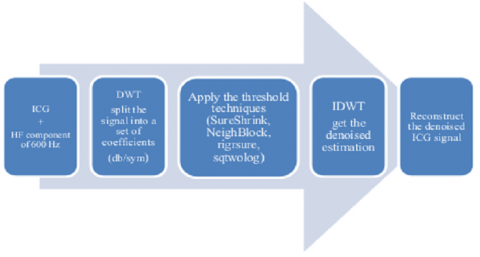Figure 2. Schematic diagram method

## 4.pngFigure 3. Interpretable diagram algorithm

2.4 Performance parameters evaluation

For the evaluation of the results of our comparisons, we based on the following parameters [24, 30], to verify the improvement of the reconstructed ICG signal: the square error (SE), the signal to noise ratio output (SNR) expressed in dB, the signal to noise ratio input (SNRi) expressed in dB which the input noise range calculate thanks to Eq. (17) is from 0 dB to 35 dB, the root mean square error (RMSE), and the percent difference root mean square (PRD) expressed in %, their formulas are defined in the order as follow:

$\mathrm{SE}=(\mathrm{x}(\mathrm{n})-\mathrm{y}(\mathrm{n}))^{2}$      (15)

$S N R=10 \log _{10}\left[\frac{\sum_{n} y^{2}(n)}{\sum_{n}(y(n)-x(n))^{2}}\quad\right]$      (16)

$\mathrm{SNR}_{\mathrm{i}}=10 \log _{10}\left[\frac{\sum_{\mathrm{n}} \mathrm{x}^{2}(\mathrm{n})}{\Sigma_{\mathrm{n}}(\mathrm{noise})^{2}}\right]$       (17)

$\operatorname{RMSE}=\frac{1}{\mathrm{~L}} \sum_{\mathrm{n}}^{\mathrm{L}}(\mathrm{x}(\mathrm{n})-\mathrm{y}(\mathrm{n}))^{2}$      (18)

$\operatorname{PRD}(\%)=100 \sqrt{\frac{\sum_{\mathrm{n}}(\mathrm{x}(\mathrm{n})-\mathrm{y}(\mathrm{n}))^{2}}{\sum_{\mathrm{n}}^{\mathrm{L}} \mathrm{x}^{2}(\mathrm{n})}}$      (19)

where, x (n) is the original signal, y (n) is the reconstructed signal, and L is the signal length.

The best denoising method has the highest SNR, the lowest PRD, the lowest RMSE and the lowest reconstructed error.

3. Results and Discussion

3.1 Results

This section presents a comparison between the selected denoising techniques. Whereas, the first purpose is to apply the linear filters. The second purpose is to denoise the ICG signal using LMS-based adaptive filters, that had chosen because it has shown reliability in studies [2, 3]. The third purpose is to choose the best threshold (SureShrink, Neighblock, Rigrsure, Sqtwolog) for two types of discrete wavelet families (Daubechies, Symlet).

Our study used different thresholds to demonstrate that the right choice of thresholding affects the obtained results' effectiveness. Hence, Neighblock never applied for ICG waveforms. Linear filters were applied to the ICG signals of 10 participants. The results are presented in Table 1.

Table 1. Estimation parameters of linear filters applied to 10 subjects

 Filters SE RMSE PRD SNR Butter 46.2660 0.2842 66.9836 4.4693 Elliptic 50.5032 0.2967 69.1668 3.8837 Gaussian 5.5373 0.0938 31.2531 13.8792 Bessel 210.0218 0.7360 185.1752 1.4735 Chebychev1 51.3537 0.2992 69.7024 3.8104 Chebychev2 198.89 0.6241 121.1752 2.321

According to the results in Table 1, the filter with the highest performance is the Gaussian filter; it has a minimum SE of about 5.5373, a minimum RMSE value of about 0.0938, a minimum PRD value of about 31.2531 and a maximum SNR of about 13.8792. Therefore, it did not fulfil the objective of our analysis for assessing accuracy. High values were obtained, hence the use of the LMS adaptive filter and discrete wavelets is of paramount importance. We added White Gaussian Noise with SNRi ranging from 0 to 35 dB to the signals to choose the best threshold technique. Figure 4a-d explains exactly the different comparisons made and the results obtained for 10 subjects. In addition, Figure 4 shows a comparison between Daubechies (db) and Symlet (sym) with the order N (2, 4, 6, 8) in relation to threshold techniques. First, we found that db2 is better than db (4, 6, 8) in Sureshrink, db4 is better than db (2, 6, 8) in Neighblock, db4 is better than db (2, 6, 8) in Rigrsure, and db8 is better than db (2, 4, 6) in Sqtwolog. Second, sym2 is better than sym (4, 6, 8) in Sureshrink, sym4 is better than sym (2, 6, 8) in Neighblock, sym8 is better than sym (2, 4, 6) in Rigrsure, and sym8 is better than sym (2, 4, 6) in Sqtwolog. Thirdly, after comparing two types of wavelets in each threshold technique, we found that the best are: db4 in Neighblock, sym8 in Rigrsure, and sym 8 in Sqtwolog. Next, the results obtained were compared with the Gaussian filter and the LMS adaptive filter at different SNRi. The results presented in Figure 5a was found using parameter estimation calculations.

## 4-1.png(a)

## 4-2.png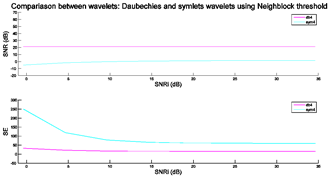(b)

## 4-3.png(c)

## 4-4.png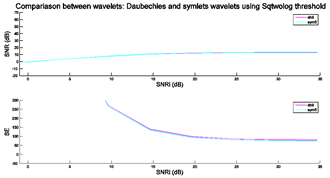(d)

Figure 4. Comparison results between different type of thresholds at different SNRi values

3.2 Discussion

This study is based on a performance study using linear filters: Bessel; Butterworth, Gaussian, Elliptic, Chebychev1, and Chebychev2 with third order; the LMS adaptive filter, as well as orthogonal wavelets such as Daubechies (db) and Symlet, used with four thresholding techniques such as Sureshrink, NeighBlock, Rigrsure, Sqtwolog. The evaluation of all comparison results is a necessary step which was carried out by calculating SE, SNR, RMSE, and PRD. In a first step, a comparison between several linear filters, the results of which are presented in Table 1. In addition, another comparison of orthogonal wavelets of different N-order (2, 4, 6, and 8) was performed.

Although the best selected wavelets were taken from the literature, namely db and sym, which are the most applied to this type of signal, they were compared with multiple thresholding techniques (Figure 4 a-d) such as NeighBlock db4, Rigrsure sym8 and Sqtwolog sym8. Next, they were also compared with the best linear filter which is the Gaussian filter and the LMS adaptive filter. The results show that the best one has a minimum value of PRD, RMSE, SE, and it has the maximum value of SNR (Figure 5a, b). According to the results obtained in the simulation, the best denoising method for the ICG signal that preserves the characteristics of the original waveform is the rigidity at level 5 of the symm8 wavelet with minimal degradation of the ICG signal shape. Figures 6 and Figure 7 show all reconstructed samples for all subjects.

The advantage of this performance study is to reduce the noise and artefacts, which cause distortions in the ICG wave, to a maximum and to preserve the shape of our ICG signal, i.e. the peak max (dZ/ dt)max of the ICG signal which is present at point C which is important in clinical decision making and monitoring of cardiovascular diseases. The peak amplitude C of the original signal and the reconstructed signal after using each denoising technique was calculated to evaluate the results. Pan-Tompkins algorithm  was used to detect the C peak. Table 2 lists the results obtained.

According to the results in the above Table 2, the best denoising method is the one that preserve the C peak amplitudes with minimal degradation. We observe that the mean C peak amplitudes of Rigrsure (sym8) equal to the mean C peak amplitudes of the original signal is about 4.716 Ohms. Moreover, for Gaussian filter is about 3.842 Ohms, 4 Ohms for LMS, 4.087 Ohms for Neighblock and 4,286 Ohms for Sqtwolog (sym8).

The results of the mean error rate listed in Table 3 provide better accuracy than the Ridder  and Chabchoub  methods at different SNRi, especially for the best thresholding technique, the Rigrsure of sym 8. For an SNRi of 0 dB, the minimum MER value for the Chabchoub and Ridder methods is equal to 0.3% and 7.3%, respectively. For 10dB, the minimum MER value for the Chabchoub and Ridder methods are equal to 0.01% and 0.7%, respectively. In this study, the minimum MER value is equal to 0.00006% in 0dB and 0.0001% in 10dB.

## 5.pngFigure 5. Comparison results of performance parameters evaluation for Gaussian filter, LMS adaptive filter and various wavelets thresholds at different SNRi values

## 6-1.png(a)

## 6-2.png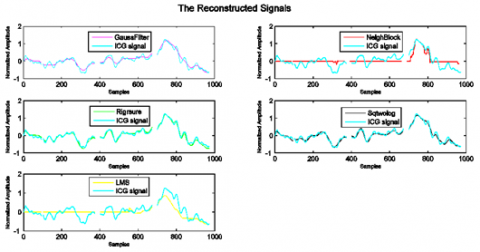(b)

Figure 6. The ICG signal reconstructed after each technique applied for subject 1

## 7.pngFigure 7. The ICG signal samples reconstructed after applying the best denoising technique for all participants: subjects 1 to 10

Table 2. Detection of peak amplitudes C (Ohms) from original noise-free ICG signals

 Participants Original C peak amplitudes C peak amplitudes after using denoising methods Gaussian filter LMS Neighblock (db4) Rigrsures (sym8) Sqwolog (sym8) P1 5021 4999 3429 4433 5001 4047 P2 4337 4337 4276 4336 4463 4743 P3 4959 2911 4049 4054 4654 4053 P4 4351 3357 4127 4353 4112 4357 P5 5401 4493 5106 4490 5287 4490 P6 5002 2511 3663 3870 5089 4294 P7 4756 3609 3383 3382 4665 3606 P8 4246 3197 4014 4424 4102 4009 P9 4486 5408 3021 4067 4365 4869 P10 4596 3395 4942 3447 4623 4396 Mean 4716 3842 4000 4087 4716 4286

Table 3. Mean error rate (%) of denoising methods for 10 subjects at different SNRi (ranging from 0 to 35 dB)

 Methods SNRᵢ 0 5 10 15 20 25 30 35 LMS 0.02855 0.02855 0.02855 0.02855 0.02855 0.02855 0.02855 0.02855 Gaussian 0.0035223 0.0041569 0.0045127 0.0047133 0.0048262 0.0048894 0.004925 0.0049452 Neighblock db4 10.1783 10.1796 10.1801 10.1803 10.1804 10.1804 10.1804 10.1805 Rigrsure sym8 6.2976e-05 8.8323e-05 0.00019118 0.00024163 0.00027019 0.000285 0.00029376 0.00029796 Sqtwolog sym 8 9.0362e-05 0.00011734 0.00021262 0.00026396 0.00029401 0.00031066 0.00032004 0.00032441
4. Conclusions

Impedance cardiography is a reliable, non-invasive and convenient method with less risk for the measurement of multiple hemodynamic parameters and it can be used by untrained personnel. It is considered a recent way to obtain results with the same or higher accuracy than invasive methods such as thermodilution, Fick or Doppler echocardiography. The ICG method is promising in monitoring cardiac contraction and functional status, and is therefore very useful for the diagnosis of cardiovascular disorders and continuous monitoring in the medical field. In this paper, a comparison was made between the LMS adaptive filter, the Gaussian filter and wavelet families (Daubechies and Symlet) using different threshold techniques such as Sureshrink, NeighBlock, Rigrsure and Sqtwolog to find the best technique for denoising the ICG signal.

To our knowledge, DWT is used to remove noise from non-stationary signals that no longer require special assumptions for thresholding or denoising in general. Very interesting results were obtained by calculating the estimation parameters, which showed that the sym8 wavelet with Rigrsure thresholding is the best in terms of noise reduction compared to other techniques.

For future work, the denoising step will be added to the current proposal, as it can help us to derive meaningful information that will be used to develop cardiography impedance systems for the non-invasive diagnosis and medical monitoring of patients.

Acknowledgment

The authors would like to thank the Directorate-General of Scientific Research and Technological Development (Direction Générale de la Recherche Scientifique et du Développement Technologique, DGRSDT, URL: www.dgrsdt.dz.

Nomenclature
 A Atrial wave B Valve opening C Maximum aortic flow rate (dZ/ dt)max cᵢ Low frequencies CO Cardiac output, L/min CWT Continuous wavelet transformation (dZ/ dt)max Maximum of the first derivate dᵢ High frequencies DWT Discrete wavelet transformation e(n) Error ECG Electrocardiogram EEG Electroencephalogram IDWT Inverse discrete wavelet transformation K Input sample length L Signal length LVET Left ventricle ejection time MER Mean error rate, % N Order Nᵢ Length of the noisy signal nth Weight coefficient vector O Mitral valve opening PRD Percent difference root mean square, % RMSE Root mean square error SE Square error SNR Noise ratio output, dB SNRi Noise ratio input, dB SURE Stein's unbiased risk principle SV Stroke volume, ml X Aortic valve closure x[n] Input signal Y Pulmonary valve closing y(n) Reconstructed signal Z Impedance Z₀ Basic thoracic impedance $V_{c}$ Intrathoracic blood volume, mL Greek symbols $\psi(.)$ Mother wavelet λ Threshold level $\theta_{i}$ Mean absolute deviation $\theta$ Standard deviation of noisy signal $\theta^{2}$ Variance of the extended block Nᵢ Length of the noisy signal $\omega$ Wavelet coefficient $\omega_{\mathrm{a}}$ Coefficient wavelet square $\beta_{\mathrm{i} \mathrm{j}}$ Shrinkage factor μ Predefined step size Subscripts i Block j Level
References

 Mansouri, S., Alhadidi, T., Chabchoub, S., Salah, R.B. (2018). Impedance cardiography: Recent applications and developments. Biomedical Research, 29(19): 3542-3552. https://doi.org/10.4066/biomedicalresearch.29-17-3479

 Hu, X., Chen, X., Ren, R., Zhou, B., Qian, Y., Li, H., Xia, S. (2014). Adaptive filtering and characteristics extraction for impedance cardiography. Journal of Fiber bioengineering and Informatics, 7(1): 81-90. https://doi.org/10.3993/jfbi03201407

 Rahman, Z.U., Mirza, S.S., Krishna, K.M. (2019). Adaptive noise cancellation techniques for impedance cardiography signal analysis. International Journal of Innovative Technology and Exploring Engineering (IJITEE), 8(9): 2278-3075. https://doi.org/10.35940/ijitee.I7531.078919

 Nabian, M., Nouhi, A., Yin, Y., Ostadabbas, S. (2017). A biosignal-specific processing tool for machine learning and pattern recognition. In 2017 IEEE Healthcare Innovations and Point of Care Technologies (HI-POCT), pp. 76-80. https://doi.org/10.1109/HIC.2017.8227588

 Hadaś-Dyduch, M. (2015). Wavelets in the prediction of short-time series. Mathematical Economics, 11(18): 43-54.

 Wahab, M.F., O’Haver, T.C. (2020). Wavelet transforms in separation science for denoising and peak overlap detection. Journal of Separation Science, 43(9-10): 1998-2010. https://doi.org/10.1002/jssc.202000013

 Sebastian, T., Pandey, P.C., Naidu, S.M.M., Pandey, V.K. (2011). Wavelet based denoising for suppression of respiratory and motion artifacts in impedance cardiography. In 2011 Computing in Cardiology, pp. 501-504.

 Salah, I.B., Ouni, K. (2017). Denoising of the impedance cardiographie signal (ICG) for a best detection of the characteristic points. In 2017 2nd International Conference on Bio-engineering for Smart Technologies (BioSMART), pp. 1-4. https://doi.org/10.1109/BIOSMART.2017.8095347

 De Ridder, S., Neyt, X., Pattyn, N., Migeotte, P.F. (2011). Comparison between EEMD, wavelet and FIR denoising: Influence on event detection in impedance cardiography. In 2011 Annual International Conference of the IEEE Engineering in Medicine and Biology Society, pp. 806-809. https://doi.org/10.1109/IEMBS.2011.6090184

 Choudhari, P.C., Panse, D.M. (2015). Denoising of radial bioimpedance signals using adaptive wavelet packet transform and Kalman filter. IOSR J VLSI Signal Process, 5: 1-8.

 Chabchoub, S., Mansouri, S., Salah, R.B. (2016). Impedance cardiography signal denoising using discrete wavelet transform. Australasian Physical & Engineering Sciences in Medicine, 39(3): 655-663. https://doi.org/10.1007/s13246-016-0460-z

 Shoemaker, W.C., Wo, C.C., Bishop, M.H., Appel, P.L., Van de Water, J.M., Harrington, G.R., Patil, R.S. (1994). Multicenter trial of a new thoracic electrical bioimpedance device for cardiac output estimation. Critical Care Medicine, 22(12): 1907-1912. https://doi.org/10.1097/00003246-199412000-00004

 AlMahamdy, M., Riley, H.B. (2014). Performance study of different denoising methods for ECG signals. Procedia Computer Science, 37: 325-332. https://dx.doi.org/10.1016/j.procs.2014.08.048

 Cordero, E., Rodino, L. (2020). Time-Frequency Analysis of Operators. De Gruyter. https://doi.org/10.1515/9783110532456

 Donoho, D.L., Johnstone, I.M. (1995). Adapting to unknown smoothness via wavelet shrinkage. Journal of the American Statistical Association, 90(432): 1200-1224. https://doi.org/10.2307/2291512

 Lye, M., Vargas, E. (1981). An analysis of impedance cardiography in the elderly. Journal of Medical Engineering & Technology, 5(6): 289-292. https://doi.org/10.3109/03091908109009363

 Yazdanian, H., Mahnam, A., Edrisi, M., Esfahani, M.A. (2016). Design and implementation of a portable impedance cardiography system for noninvasive stroke volume monitoring. Journal of Medical Signals and Sensors, 6(1): 47.

 Bernstein, D.P. (2010). Impedance cardiography: Pulsatile blood flow and the biophysical and electrodynamic basis for the stroke volume equations. Journal of Electrical Bioimpedance, 1(1): 2-17. https://doi.org/10.5617/jeb.51

 Bernstein, D.P., Lemmens, H.J.M. (2005). Stroke volume equation for impedance cardiography. Medical and Biological Engineering and Computing, 43(4): 443-450. https://doi.org/10.1007/BF02344724

 Stevanovic, P., Scepanovic, R., Radovanovic, D., Bajec, D., Perunovic, R., Stojanovic, D., Stevanovic, D. (2008). Thoracic electrical bioimpedance theory and clinical possibilities in perioperative medicine. Signa vitae: journal for Intesive Care and Emergency Medicine, 3(Suppl. 1): 22-27. https://doi.org/10.22514/SV31.022008.5

 Summers, R.L., Shoemaker, W.C., Peacock, W.F., Ander, D.S., Coleman, T.G. (2003). Bench to bedside: electrophysiologic and clinical principles of noninvasive hemodynamic monitoring using impedance cardiography. Academic Emergency Medicine, 10(6): 669-680. https://doi.org/10.1197/aemj.10.6.669

 Podtaev, S., Stepanov, R., Dumler, A., Chugainov, S., Tziberkin, K. (2012). Wavelet analysis of the impedance cardiogram waveforms. In Journal of Physics: Conference Series, 407(1): 012003. https://doi.org/10.1088/1742-6596/407/1/012003

 Dautov, Ç.P., Özerdem, M.S. (2018). Wavelet transform and signal denoising using Wavelet method. 2018 26th Signal Processing and Communications Applications Conference (SIU), pp. 1-4. https://doi.org/10.1109/SIU.2018.8404418

 Khiter, A., Adamou-Mitiche, A.B., Mitiche, L. (2020). Denoising electrocardiogram signal from electromyogram noise using adaptive filter combination. Revue d'Intelligence Artificielle, 34(1): 67-74. https://doi.org/10.18280/ria.340109

 Antoniadis, A., Bigot, J., Sapatinas, T. (2001). Wavelet estimators in nonparametric regression: A comparative simulation study. Journal of Statistical Software, 6, pp-1.

 Cohen, L. (1989). Time-frequency distributions-a review. Proceedings of the IEEE, 77(7): 941-981. https://doi.org/10.1109/5.30749

 Cohen, R. (2012). Signal denoising using wavelets. Project Report. Department of Electrical Engineering Technion, Israel Institute of Technology, Haifa.

 Cai, T.T., Silverman, B.W. (2001). Incorporating information on neighbouring coefficients into wavelet estimation. Sankhyā: The Indian Journal of Statistics, Series B, 127-148. https://www.jstor.org/stable/25053168

 Roulias, D., Loutas, T., Kostopoulos, V. (2013). A statistical feature utilising wavelet denoising and neighblock method for improved condition monitoring of rolling bearings. Chemical Engineering Transactions, 33: 1045-1050. https://doi.org/10.3303/CET1333175

 Cai, T.T. (1999). Adaptive wavelet estimation: A block thresholding and oracle inequality approach. The Annals of Statistics, 27(3): 898-924. https://doi.org/10.1214/aos/1018031262

 Pan, J., Tompkins, W.J. (1985). A real-time QRS detection algorithm. IEEE Transactions on Biomedical Engineering, (3): 230-236. https://doi.org/10.1109/TBME.1985.325532